# Homomorphisms

## Cores of Cayley graphs ★★

Author(s): Samal

Conjecture   Let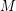be an abelian group. Is the core of a Cayley graph (on some power of) a Cayley graph (on some power of)?

Keywords: Cayley graph; core

## Pentagon problem ★★★

Author(s): Nesetril

Question   Letbe a 3-regular graph that contains no cycle of length shorter than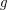. Is it true that for large enough~there is a homomorphism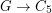?

Keywords: cubic; homomorphism

## Mapping planar graphs to odd cycles ★★★

Author(s): Jaeger

Conjecture   Every planar graph of girth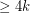has a homomorphism to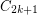.

Keywords: girth; homomorphism; planar graph

## Weak pentagon problem ★★

Author(s): Samal

Conjecture   Ifis a cubic graph not containing a triangle, then it is possible to color the edges ofby five colors, so that the complement of every color class is a bipartite graph.

## Algorithm for graph homomorphisms ★★

Author(s): Fomin; Heggernes; Kratsch

Question

Is there an algorithm that decides, for input graphsand, whether there exists a homomorphism fromtoin timefor some constant?

## Circular choosability of planar graphs ★

Author(s): Mohar

Let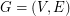be a graph. Ifandare two integers, a-colouring ofis a functionfrom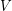tosuch thatfor each edge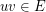. Given a list assignment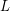of, i.e.~a mapping that assigns to every vertexa set of non-negative integers, an-colouring ofis a mappingsuch that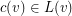for every. A list assignmentis a--list-assignment if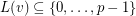andfor each vertex. Given such a list assignment, the graph G is--colourable if there exists a--colouring, i.e.is both a-colouring and an-colouring. For any real number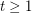, the graphis--choosable if it is--colourable for every--list-assignment. Last,is circularly-choosable if it is--choosable for any,. The circular choosability (or circular list chromatic number or circular choice number) of G is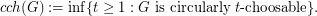Problem   What is the best upper bound on circular choosability for planar graphs?

Keywords: choosability; circular colouring; planar graphs